# Finding the Angles of the Truncated Icosahedron

2011 October 08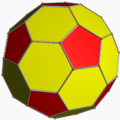Truncated Icosahedron

I’ve been interested in various geometric shapes for many years, from the simple cubes, prisms, and pyramids made in middle school, to the geodesic domes used to build houses.

I have cut and glued numerous pieces of paper into just about all of the regular polyhedra.

One project I have wanted to do for some time is to build a wire-frame truncated icosahedron, otherwise known as a buckyball or the general shape of a soccer ball. Folding paper and gluing the edges where they meet is a easy task, even for kids. Gluing wood dowels or soldering wire together at specific angles tends to be a wee bit more complicated, especially if you don’t know what the angles should be.

For any of the regular polyhedra, there are hundreds of internet sites and dozens of books that will detail out how to find the dihedral angle for each face-face edge. The trouble is, wire frames don’t have “faces,” each edge joins two or more edges at a point and spread out at equal or unequal angles that are never in the same plane. I have searched high and low, but have not yet found any website that gives these angles.

I have taken the painful steps of calculating these angles for the truncated icosahedron. Using these steps you can then find the angle for any of the other regular polyhedra.

First, let’s define a few items. The truncated icosahedron is made up of 12 pentagons and 20 hexagons, all with the same side length. Since they all have the same size sides, we will define every other measurement in terms of side length, and call it 1. It can be 1 inch, 1 cm, 1 mile, or 1 light-year, it doesn’t matter.

Each pentagon is surrounded by 5 hexagons. Each hexagon is surrounded by 3 pentagons and 3 hexagons, alternating. We will call each edge where a pentagon meets a hexagon a HP edge and where 2 hexagons meet, we’ll call a HH edge. Every edge will join with two others, always an HH edge and two HP edges will meet at each vertex.

The dihedral (face to face) angle for the HH edges is given as: $\cos^{-1}\left(\Large{-\frac{\sqrt{5}}{3}}\right) = 138.189685\ldots^\circ$.

The dihedral angle for the HP edges is: $\cos^{-1}\left(\Large{-\sqrt{\frac{5+2\sqrt{5}} {15} } }\right) = 142.622632\ldots^\circ$.

As I said, there are lots of sources to find the breakdown of the math used to get these results. I only add them here for your convenience.

The diameter can be “unrolled” to show there is a repeat (Fig 1) from P1 to P2 to P3. P3 would be the same point as P1.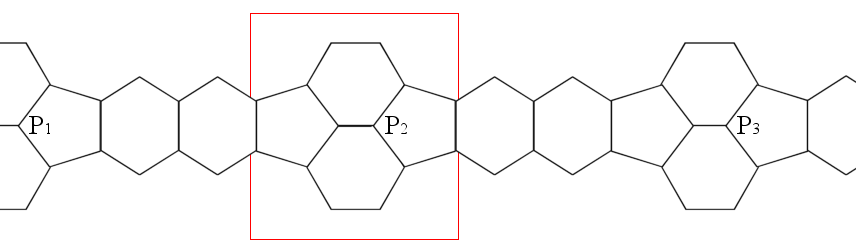This makes a decagon (10-sided figure), traveling across the faces of 4 pentagons, 4 hexagons, and 2 HH edges. This gives us a decagon with 4 HP angles and 2 HH angles, but we don’t know the other 4 Pentagon-to-Pentagon (Pent-Pent) edge angles.

All decagons have a total interior angle measurement of 1440°, using the formula for any N-gon, (N-2)x180. If we then use a little algebra, we get:

1440 = 4 * HP angle + 2 * HH angle + 4 * Pent-Pent edge angle

Substituting known values:

1440 = 4 x 142.622632 + 2 x 138.189685 + 4 x Pent-Pent edge angle
1440 = 570.490528 + 276.379370 + 4 x Pent-Pent edge angle
1440 = 846.869898 + 4 x Pent-Pent edge angle
1440 - 846.869898 = 4 x Pent-Pent edge angle
593.130102        = 4 x Pent-Pent edge angle
148.2825255       = Pent-Pent edge angle

So if I wish to create a wire-frame of a truncated icosahedron, then I just need to join two wires at 108°, the interior angle for a pentagon, and add a third sticking straight out and rising up at about an angle of 31¾° (180°-148¼°).

But, is this correct? How can it be verified? Is there a way to find “exact” measurements?

## Finding the Angles of a Pentagon-Hexagon-Hexagon Vertex

Taking the red-outlined area from Fig 1, and rotating we get Fig 2. Note, these figures are not to scale or proportion.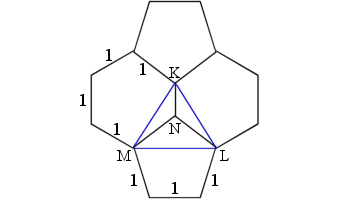Figure 2

Drawing (blue) lines across the widths of the hexagons (KL & KM) and the pentagon (ML), we then get a pyramid with base ΔKLM and apex N (Fig 3).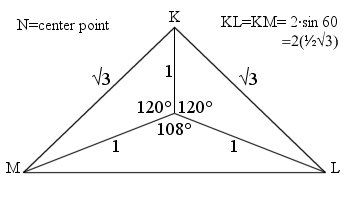Figure 3

Looking at ∠KNL and ∠KNM, each is 120° and ∠MNL=108°. The ΔNKL (Fig 4) shows how we get KL & KM from 30-60-90 triangles, each is $2\cdot \sin60^\circ = 2·\frac{\sqrt3}{2} = \sqrt3$.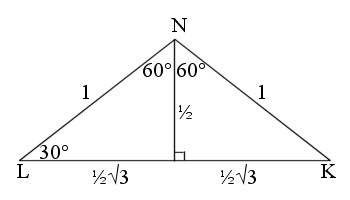Figure 4

ΔNML (Fig 5) shows the length for LM, we don’t need exact figures, 2·sin 54° will do for now. Lines GL & GM are sin 54°.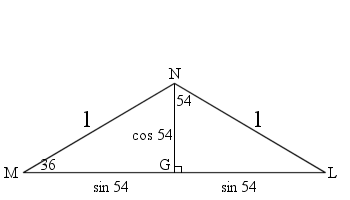Figure 5

ΔKML (Fig 6) uses the Pythagorean Theorem to find length of line KG. We don’t need to find the actual length, as we are about to square it anyway.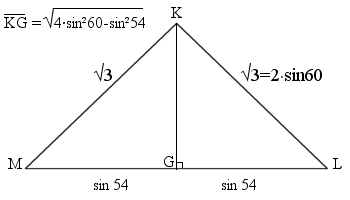Figure 6

ΔNKG (Fig 7) shows the angle η the we are looking for. Note: I drew this image even though knew that η was obtuse. 🙁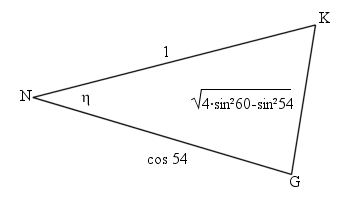Figure 7

Using the Law of Cosines, we get:

\begin{align}\cos\eta &= \frac{1^2 + \color{Blue}{NG^2} + \color{LimeGreen}{KG^2}}{2\cdot1\cdot \color{Blue}{NG}}\\ &= \frac{1 + \color{Blue}{cos^2(54)} – \color{LimeGreen}{\bigl [4\cdot sin^2(60) – sin^2(54)\bigr ]}}{2\cdot1\cdot \color{Blue}{cos (54)}}\\ &= \frac{1 + \color{Blue}{cos^2(54)} \color{LimeGreen}{- 4\cdot sin^2(60)} + \color{LimeGreen}{sin^2(54)}}{2\cdot \color{Blue}{cos (54)}} & \text{Factor inside the brackets}\\ &= \frac{1 + \color{red}[\color{Blue}{cos^2(54)} + \color{LimeGreen}{sin^2(54)}\color{red}] \color{LimeGreen}{- 4\cdot sin^2(60)}}{2\cdot \color{Blue}{cos (54)}} & \text{and rearrange}\\ &= \frac{1 + \color{red}1 – 4\cdot sin^2(60)}{2\cdot \color{Blue}{cos (54)}} & \text{Using the identity cos² α + sin² α = 1}\\ &= \frac{2 – 4\cdot sin^2(60)}{2\cdot \color{Blue}{cos (54)}}\\ &= \frac{\color{yellow}{1 – 2\cdot sin^2(60)}}{\color{Blue}{cos (54)}} & \text{factor out 2}\\ &= \frac{\color{yellow}{\cos(2\cdot 60)}}{\color{Blue}{cos (54)}} & \text{Using the identity cos 2α = 1 – 2·sin² α}\\ &= \frac{\color{yellow}{cos(120)}}{\color{Blue}{cos(54)}}\\ &= \frac{\color{yellow}{-\frac12}} {\color{Blue}{\sqrt{\frac{5-\sqrt{5}} {8} } }} & \text{Substitute values}\\ &= \frac{-1}{2} \cdot \frac{\sqrt{8}}{\sqrt{5-\sqrt{5}}} & \text{invert}\\ &= \frac{-2\sqrt2}{2\sqrt{5-\sqrt{5}}}\cdot \frac{\sqrt{5+\sqrt{5}}}{\sqrt{5+\sqrt{5}}} & \text{Multiply by conjugate}\\ &= \frac{-\sqrt2\sqrt{5+\sqrt{5}}}{\sqrt{25-5}}\\ &= \frac{-\sqrt2\sqrt{5+\sqrt{5}}}{\sqrt{20}}\\ &= \frac{-\sqrt2\sqrt{5+\sqrt{5}}}{\sqrt{4\cdot 5}}\\ &= \frac{-\sqrt2\sqrt{5+\sqrt{5}}}{2\sqrt5}\\ &= \frac{-\sqrt2\sqrt{5+\sqrt{5}}}{2\sqrt5}\cdot \frac{\sqrt5}{\sqrt5}\\ &= \frac{-\sqrt2\sqrt5\sqrt{5+\sqrt{5}}}{20}\\ &= -\sqrt{\frac{5+\sqrt5}{10} } \end{align}
∠η = 148.2825255…°

Hooray! It matches the value we found earlier.

## Finding the Angles of a Regular Pentagonal Pyramid

A truncated icosahedron is made by truncating (cutting off) a regular pentagonal pyramid from each of the tips of an icosahedron, hence the name.

The angle of the pentagon face to any of the triangle edges will be 180° minus the Pent-Pent edge angle.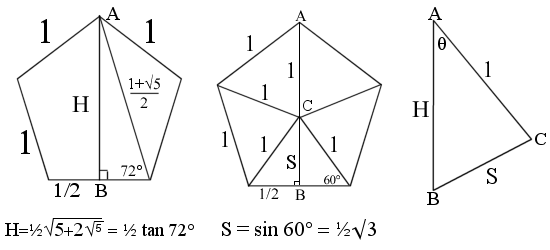Figure 8a, 8b, 8c

In order to find the angles we want, we must first find the height of a pentagon (Fig 8a). Either use the Pythagorean Theorem or trigonometry to find height H.

The faces of the pyramid are all equilateral triangles. Finding the triangle height S is elementary (Fig 8b). Side note: S is 1/3 the length of the original icosahedron.

Slicing through the pyramid through points A, B, and C, reveals (Fig 8c) ΔABC.

We then use the Law of Cosines to find ∠θ.

\begin{align}\cos \theta &= \frac{1^2 + \color{skyblue}{H^2} – \color{limegreen}{S^2}}{2\cdot1\cdot \color{skyblue}H}\\ &= \frac{1^2 + \color{skyblue}{\frac14\cdot tan^2(72)} \color{limegreen}{-\frac34}}{2\cdot1\cdot \color{skyblue}{\frac12\cdot tan(72)}}\\ &= \frac{\frac44 \color{limegreen}{-\frac34} + \color{skyblue}{\frac14\cdot(5+2\sqrt{5})} }{2\cdot \color{skyblue}{\frac12\sqrt{5+2\sqrt{5}}}} \\ &= \frac{\frac14+\frac14(5+2\sqrt5)}{\sqrt{5+2\sqrt5}} \\ &= \frac{1+\quad 5+2\sqrt5}{4\sqrt{5+2\sqrt5}}\\ &= \frac{6+2\sqrt5}{4\sqrt{5+2\sqrt5}}\\ &=\frac{3 + \sqrt{5}} {2\sqrt{5 + 2\sqrt{5}}}\\ &=\frac{3 + \sqrt{5}} {2\sqrt{5 + 2\sqrt{5}}} \cdot \frac{\sqrt{5 – 2\sqrt{5}}}{\sqrt{5 – 2\sqrt{5}}} & \text{multiply by conjugate}\\ &=\frac{(3 + \sqrt{5})\sqrt{5-2\sqrt5}} {2\sqrt{25-20}} \\ &=\frac{\color{yellow}{(3 + \sqrt{5})}\sqrt{5-2\sqrt5}} {2\sqrt5} \\ &=\frac{\color{yellow}{\sqrt{14 + 6\sqrt{5}}}\sqrt{5-2\sqrt5}} {2\sqrt5} & \color{yellow}{(3 + \sqrt{5})^2 = 14+6\sqrt5}\\ &=\frac{\sqrt{70 – 60 + 30\sqrt5 -28\sqrt5}} {2\sqrt5} \\ &=\frac{\sqrt{10 + 2\sqrt5}} {2\sqrt5} \\ &=\frac{\sqrt{10 + 2\sqrt5}} {2\sqrt5} \cdot \frac{\sqrt5}{\sqrt5} \\ &=\frac{\sqrt{50 + 10\sqrt5}} {10} \\ &=\frac{\sqrt{10}\sqrt{5 + \sqrt5}} {10} \\ &=\sqrt{\frac{5 + \sqrt5}{10}}\end{align}

∠θ = 31.7174744…°

θ + η = 180°

$\cos \theta = -\cos \mu$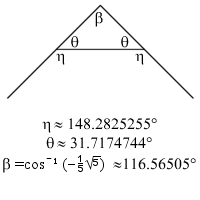Figure 9

A double verified result.

Also, now that I know these two angles, I see that (180° – 2·θ) is the dihedral angle (β) for the dodecahedron and the truncated dodecahedron, as seen in the triangle in Figure 9.